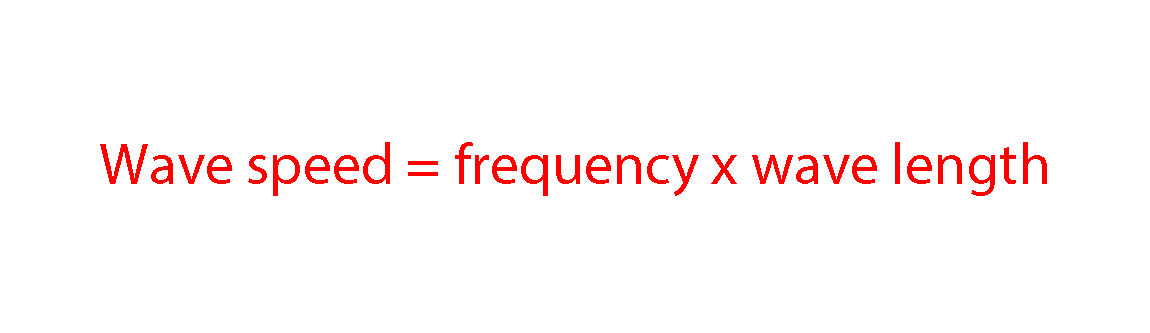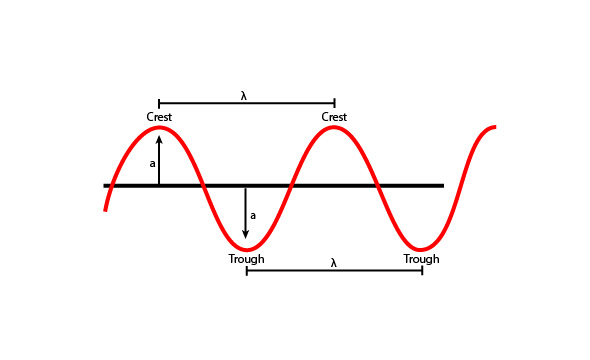# Wave speed

Wave speed is the speed (velocity) at which a wave is travelling.

The formula for calculating wave speed is:Wave speed = f λ  or  v = f λSpeeding along she frequently (frequency) waved at length.

NOTE:

This does not only relate to waves in water but all waves, including light waves and electromagnetic waves.

v = f λ where:

v = wave speed (measured in metres per second – m/s)

f = frequency (measured in hertz – Hz)

λ (pronounced lambda) = wavelength (measured in metres)Example 1

A wave at sea travels with a velocity of 25m/s. If it has a wavelength of 10m, what is its frequency?

Wave speed = frequency x wave length

Divide both sides by wavelength to make frequency the subject,

\frac{Wave\ speed}{wavelength}\ =\ \frac{\strike{wavelength\ }\times frequency}{\strike{wavelength}}

Frequency\ =\frac{Wave\ speed\ }{wavelength}=\ \frac{25}{10}\ =\ 2.5Hz

Example 2

Lizzy reads the back label of her microwave oven. It says frequency = 2,450MHz. If the speed of microwaves is 3.00 x 108 m/s, what wavelength are they?

The first thing you must do to answer this question is convert the frequency to the standard units, which means converting from MHz to Hz (1 MHz = 106 Hz). So 2,450 MHz = 2,450 x 106 Hz.

Wave speed = frequency x wave length

Divide both sides by frequency to make wavelength the subject:

\frac{Wave\ speed}{frequency}\ =\ \frac{\strike{frequency}\ \times wavelength}{\strike{frequency}}

wavelength=\frac{Wave\ speed\ }{frequency}=\frac{3\ \times10^{^8}}{2,450\ \times10^6}\ =\ 0.122m

0.122m = 12.2cm

Example 3

An electromagnetic wave travels at a speed of 300,000,000 m/s and has a wavelength of 15cm. Calculate the frequency of the electromagnetic wave.

Wave speed = frequency x wave length

Divide both sides by wavelength to make frequency the subject,

\frac{Wave\ speed\ }{wavelength}=\ \frac{\strike{wavelength}\times frequency}{\strike{wavelength}}

Frequency\ =\frac{\ Wave\ speed}{wavelength}

However the wavelength given in the question is not in the standard unit and so must first be converted from cm to metres.

15cm = 0.15m So:

Frequency\ =\ \frac{Wave\ speed\ }{wavelength}=\frac{300,000,000}{0.15}=2,000,000,000\ Hz\ =\ 2GHz

NOTE:

We have converted the answer to GHz in this case. There are 1 billion Hz (109) in 1 GHz, so 2 billion Hz = 2GHz.

Example 4

A TV station broadcasts at 500,000 kHz. The waves travel through the air at 300,000,000 m/s. Calculate the wavelength of the waves broadcast by this station.

Wave speed = frequency x wave length

Divide both sides by frequency to make wavelength the subject,

\frac{Wave\ speed\ }{frequency}\ =\ \frac{\strike{frequency}\ \times\ wavelength}{\strike{frequency}}

Wavelength\ =\ \frac{Wave\ speed}{frequency}

Beware, the question gives the frequency in kHz so you will need to convert this to Hz before you can put the figures into the formula.

500,000 kHz = 500,000,000 Hz So:

Wavelength\ =\frac{\ 300,000,000\ }{500,000,000}=\ 0.6m